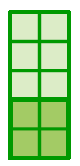### Home > CC1MN > Chapter 3 > Lesson 3.1.2 > Problem3-31

3-31.

For each of the following portions, draw a diagram of the mixture in the jar. Then shade a layer that would correspond to this portion of raisins. Finally, order these portions from least to greatest.

1. $40\%$

1. $\frac { 1 } { 4 }$

1. $25\%$

1. $\frac { 1 } { 3 }$

Use the percent rulers below to help understand how the portions in parts (a) to (d) compare. Can this help you represent the portions in diagrams? Two percent rulers. The percent ruler on the left is scaled by 10%. The beginning, middle, and end are labeled 0%, 50%, and 100% respectively. The percent ruler on the right is scaled by 25% and labeled 0%, 25%, 50%, 75%, and 100%.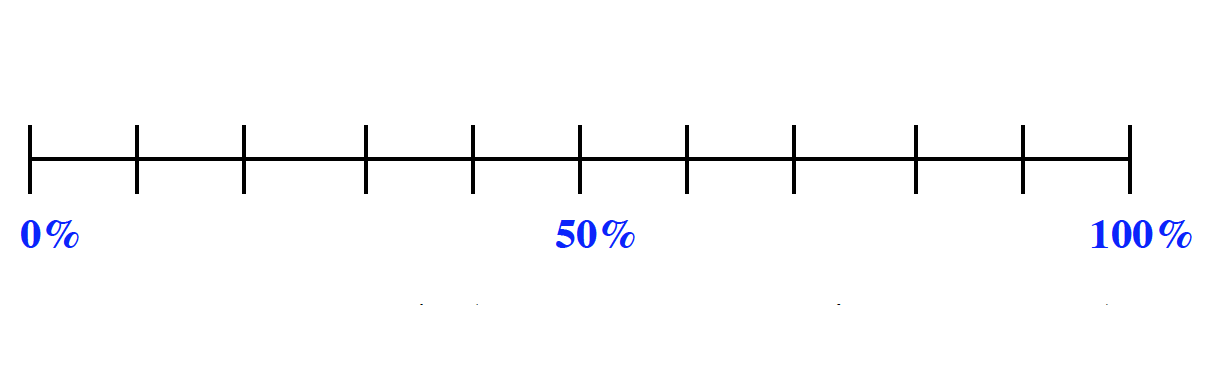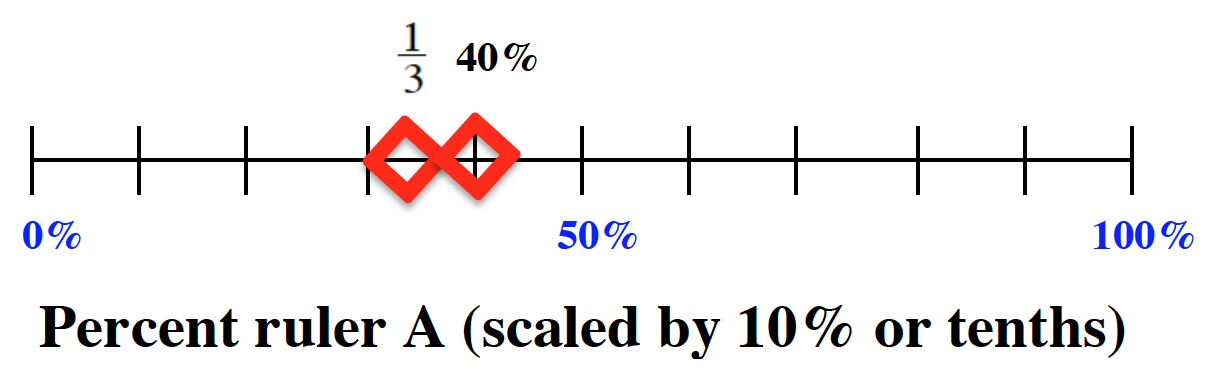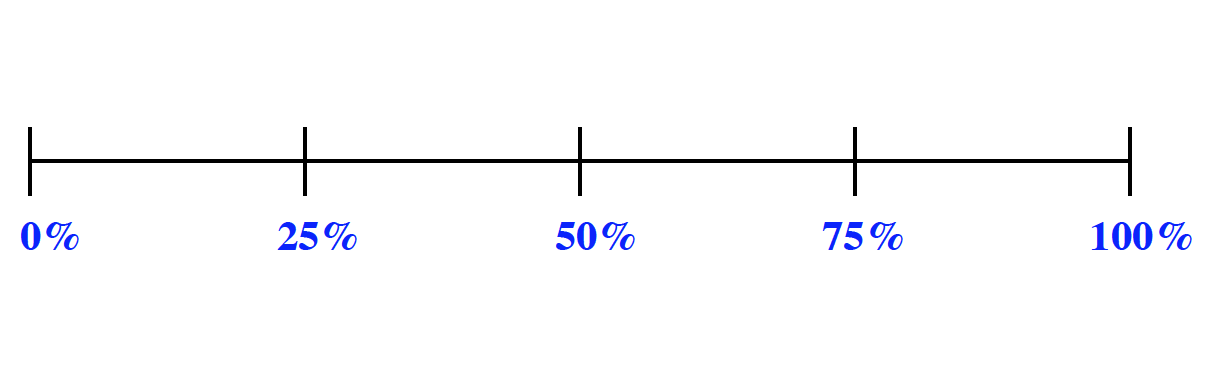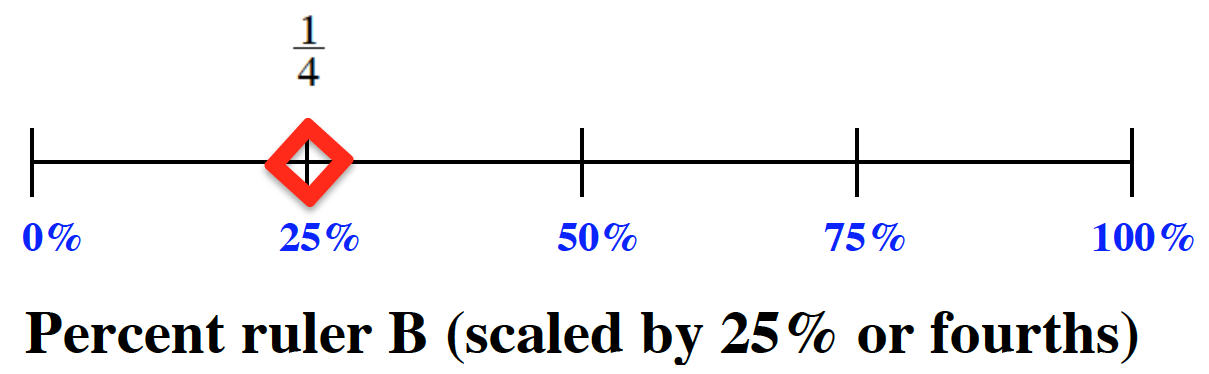Chloe found and marked $40\%$ on the ruler. She knew that $40\%$ is the same as four tenths, so she drew the picture on the right. Can you find the rest of the portions and draw diagrams to represent them?

The rest of the portions are marked on the percentage rulers. Can you list them in order from least to greatest? The percent ruler scaled by 10% has added: one third, a little after the third mark , and 40%, on the fourth mark. The percent ruler scaled by 25% has added 1 fourth, above the 25%.

$\text{Note that 25% and }\frac{1}{4}\text{ are equal.}$

Don't forget to draw your diagrams!